# Spark2.0机器学习系列之11： 聚类(幂迭代聚类， power iteration clustering， PIC)spark 同时被 2 个专栏收录22 篇文章 1 订阅

在Spark2.0版本中（不是基于RDD API的MLlib），共有四种聚类方法：
（1）K-means
（2）Latent Dirichlet allocation (LDA)
（3）Bisecting k-means（二分k均值算法）
（4）Gaussian Mixture Model (GMM)。
基于RDD API的MLLib中，共有六种聚类方法：
（1）K-means
（2）Gaussian mixture
（3）Power iteration clustering (PIC)
（4）Latent Dirichlet allocation (LDA)**
（5）Bisecting k-means
（6）Streaming k-means
多了Power iteration clustering (PIC)和Streaming k-means两种。
本文将PIC,即幂迭代聚类。其它方法在我Spark机器学习系列里面都有介绍。

# 概述

谱聚类（Spectral Clustering）相信大家可能非常熟悉（如果不熟悉的话相关资料也比较多），而幂迭代聚类（Power iteration clustering）则也许会比较陌生。其实这两者之间有很多相似的地方，但是算法还是有较大差异的，这些后面会慢慢道来。
幂迭代聚类来自Frank Lin 和William W. Cohen这两位卡内基梅隆大学大牛，发表于ICML 2010。深入了解算法则需要看看大牛的原文。
这里给出链接http://www.cs.cmu.edu/~wcohen/postscript/icml2010-pic-final.pdf
如果具备一定的谱聚类和Graphx图计算的知识，就比较容易理解幂迭代聚类，不过不太懂也没有关系，Graphx图计算可以简单看看我的Spark系列博文，谱聚类后面会简单介绍一下，另外算法的数学基础是幂迭代求特征值，我后面会详细介绍。
两位大牛提出一种简单且可扩展的图聚类方法，称之为幂迭代聚类（PIC）。在数据归一化的逐对相似矩阵上，使用截断的幂迭代，PIC寻找数据集的一个超低维嵌入（低纬空间投影，embedding ），这种嵌入恰好是很有效的聚类指标，使它在真实数据集上总是好于广泛使用的谱聚类方法（比如说NCut）。PIC在大数据集上非常快，比基于当前（2010年）最好的特征向量计算技术实现的NCut还要快1000倍。
We present a simple and scalable graph clustering method called power iteration clustering (PIC). PIC finds a very low-dimensional embedding of a dataset using truncated power iteration on a normalized pair-wise similarity matrix of the data. This embedding turns out to be an effective cluster indicator, consistently outperforming widely used spectral methods such as NCut on real datasets. PIC is very fast on large datasets, running over 1,000 times faster than an NCut implementation based on the state-of-the-art IRAM eigenvector computation technique.

# 幂迭代法求矩阵的主特征值

首先还是理清基本的数学算法，这样后面分析就容易多了。“幂迭代”法求特征值，也有直接就叫做“幂法”求特征值的，也是最基础的一种特征值迭代法求解方法。
适合计算大型稀疏矩阵的主特征值，即按模最大的特征值，同时也得到了对应的特征向量（这不就是为大数据集，通常还是稀疏矩阵量身打造的吗？呵呵）。
它的优点是方法简单，理论依据是迭代的收敛性（这两点要看完下面的过程才能深刻的理解）

幂法基本思想是:若我们求某个n阶方阵 A $A$的特征值和特征向量,先任取一个非零初始向量v(0)$v^{(0)}$,进行如下的迭代计算,直到收敛（下面都是对｛ v(k)   k=0,1,... $v^{(k)} ，\ \ \ k=0,1,...$｝序列而言的）：

v(k+1)=Av(k)       k=0,1,...

当 k $k$增大时,序列的收敛情况与绝对值最大的特征值有密切关系,分析这一序列的极限,即可求出按模最大的特征值和特征向量。
假定矩阵A$A$有n个线性无关的特征向量.n个特征值按模由大到小排列:

λ1>=λ2>=>=λn      (2)

其相应的特征向量为:
e1,e2,,en      (3)

它们构成n维空间的一组正交基.任取的初始向量 v(0)) $v^{(0)})$当然可以由它们的线性组合给出：
v(0)=c1e1+c2e2++cnen      (4)

v(k)=Av(k1)=A2v(k2)==Akv(0) =c1λk1e1+c2λk2e2++cnλknen      (5)

得出公式（5），后面就好分析，公式（5）这一步可以用递推法去理解，如下：
v(0)=c1e1+c2e2++cnen v(1)=Av(0)=A(c1e1+c2e2++cnen)=c1(Ae1)+c2(Ae2)+...+cn(Aen) =c1λ1e1+c2λ2e2+...+cnλnen ... v(k)=c1λk1e1+c2λk2e2++cnλknen

下面按模最大特征值 λ1 $λ_1$是单根的情况讨论:
将公式(5)稍加变形：

v(k)=λk1(c1e1+c2(λ2λ1)ke2++cn(λnλ1)ken)   (6)

若a1≠0，由于 0<|λi/λ1|<1(i2) $0<|λ_i/λ_1 |<1 (i≥2)$,考虑到(0,1)之间的数的k次方的极限为0，因此 k $k$充分大时，
v(k)λk1c1e1

所以不难得出，当k充分大时：
v(k)λk1c1e1v(k+1)λk+11c1e1=>λ1v(k+1)v(k)

也就是说进行 v(k+1)=Av(k)k=0,1,... $v^{(k+1)}=Av^{(k)} ，k=0,1,...$迭代，当达到收敛以后，利用相邻两次迭代结果的比值就可以计算出 λ1 $λ_1$。等等，好像还是有一些问题， v(k) $v^{(k)}$是一个向量，怎么相除啊？关于这个问题，可以参考文献【6】，说的比较详细。其实一 定不能回避这个问题，就直接给出如下分量表达式
λ1v(k+1)jv(k)jjj   (7)

如果直接出给分量表达式，那么谁看得懂这个公式怎么来的， j $j$什么意思？所以简单看看文献【6】，心里就踏实多了，是分多种情况的，大家直接链接吧，有点复杂，我就不细说了。
所以我们能看出，方法确实简单，非常容易就可以写一个能运行的c/c++程序，方法的理论依据就是迭代的收敛性。利用了模大小不同的各特征值在迭代中随着迭代次数的增加贡献差异愈发明显这个特性，举个不恰当例子，就好比选一群马来长跑，其中有一匹特别能跑，而且耐力也特别好，是一匹千里马，一开有一定差距，慢慢的就把其它马落的越来越来，以至于最后大家忽略了其它的马，只看这以匹就行。
以上就是幂迭代求λ1$λ_1$的基本原理，至于对收敛速度的分析，以及各类加速方法，就不再继续了，敲公式太累了。。。（其实省略的这些内容从公式推理上来说是比较简单的！！！）

# 谱聚类

谱聚类(Spectral Clustering, SC)是一种基于图论的聚类方法——将带权无向图划分为两个或两个以上的最优子图，使子图内部尽量相似，而子图间距离尽量距离较远，以达到常见的聚类的目的。其中的最优是指最优目标函数不同，可以是割边最小分割——如图1的Smallest cut(如后文的Min cut)， 也可以是分割规模差不多且割边最小的分割——Best cut(如后文的Normalized cut)。
谱聚类能够识别任意形状的样本空间且收敛于全局最优解，其基本思想是利用样本数据的相似矩阵 L $L$(拉普拉斯矩阵)进行特征分解后得到的特征向量进行聚类。
给定样本的原始特征，我们需要计算两两样本之间的相似度值，才能构造出关联矩阵A$A$。我们一般使用高斯核函数来计算相似度。
拉普拉斯矩阵   L=DA   $\ \ L=D-A\ \$, 其中 D $D$为图的度矩阵，度是图论中的概念，也就是矩阵A$A$行或列的元素之和。谱聚类基于矩阵 L $L$来进行研究

# 幂迭代聚类

假设有数据集X=x1,x2,...,xn$X={x_1,x_2,...,x_n}$,相似函数 s(xi,xj) $s(x_i,x_j)$。定义关联矩阵 A $A$,并抽象成图。（这部分和谱聚类是一样的）。
归一化的关联矩阵W定义为：

W=D1A,D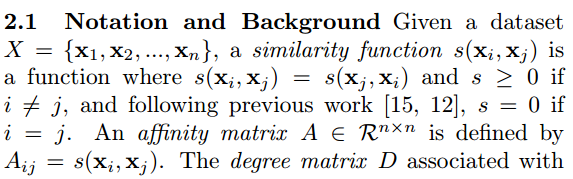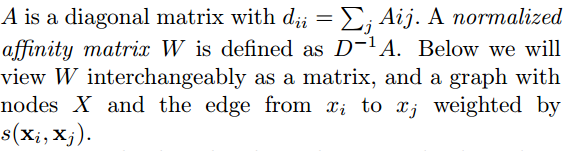我们注意到 W $W$最大的特征值是L$L$的最小特征值。而计算矩阵最大特征值的一个有效的方法是PI。

PIC算法（没有完全按照原论文书写符号，将迭代写成诸如 v(t+1)=Av(t) $v^{(t+1)}=Av^{(t)}$这样子而不是 vt+1=Avt $v^{t+1}=Av^{t}$，非常易读而不与指数混淆）：
（1）输入:一个按行归一化的关联矩阵 W $W$,和期望聚类数k$k$
（2）随机选取一个非零初始向量 v0 $v_0$
（3） v(t+1)=Wv(t)||Wv(t)||1  $v^{(t+1)}=\frac{{W v^{(t)}}}{||Wv^{(t)}||_1}\\\$       δ(t+1)=|v(t+1)v(t)| $\ \ \ \ \ \ 且令δ^{(t+1)}= |v^ {(t+1)} − v^{( t)} |$
（4）增加 t  $t\$ 重复迭代（3）式，直到 |δtδt1| 0 $|δ^{ t} − δ^{ t−1} | \simeq\ 0$ 停止
（5）使用k-means对向量 v(t) $v^{(t)}$中的点进行聚类
（6）输出：类 C1,C2,...,CK $C_1,C_2,...,C_K$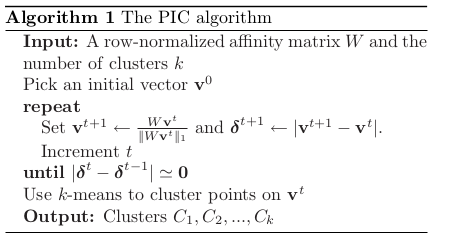如何理解上面的计算过程呢？有这么一些关键的地方：
（1）使用了所谓的早期截断(Early Stopping),简单来说就是：不像常规迭代过程一直要迭代至最终收敛，而是迭代到一定程度就停止迭代，原因如下：
作者研究发现，在使用归一化关联矩阵 W $W$矩阵运行幂指数迭代时，如果运行至最终收敛，并没有得到任何感兴趣的结果，但是发现，PI过程中产生的一些向量v  $v\ \$极其有意义！
The central observation of this paper is that, while running PI to convergence on W does not lead to an interesting result, the intermediate vectors obtained by PI during the convergence process are extremely interesting.
（2）如果进行早期截断呢？

v(k)=λk1(c1e1+c2(λ2λ1)ke2++cn(λnλ1)ken   (6)

v(t)c1λt1=e1+c2c1(λ2λ1)te2+cnc1(λnλ1)ten   (8)

很明显PI向主特征值 e1 $e_1$的收敛速度取决于 λi/λ1, i=2,...k $λ_i/λ_1,\ i=2,...k$，为什么是主要的k个特征值而不是全部的 nk<n $n个特征值呢（k，如果把 k+1,...,n $k+1,...,n$这些较小的特征值作为数据的噪音，在一定次数迭代后很快就可以忽略不计的话，是比较好理解的。研究发现，当这些噪音项去掉之后，迭代的收敛速度几乎是恒定的速度。
更具体一些，定义收敛速度为： δ(t)=|v(t)v(t1)|, $δ^{(t)}= |v^ {(t)} − v^{( t-1)} | ,收敛的加速度为：$ |δ(t)δ(t1)| $|δ^{( t)} − δ^{ (t−1}) |$，当收敛的加速度小于一个极小值时，可以认为收敛近似恒定了，那么就选择这个时候进行截断迭代过程吧（Early Stopping），至于为什么这时候是最好的时机，为什么这时候对向量 vt $v^{t}$中的点进行k-means是最好的选择，看来还需要更多的分析和实例聚类结果实验对比（这在论文里面写的都很详细），整个的计算流程前面已经给出了。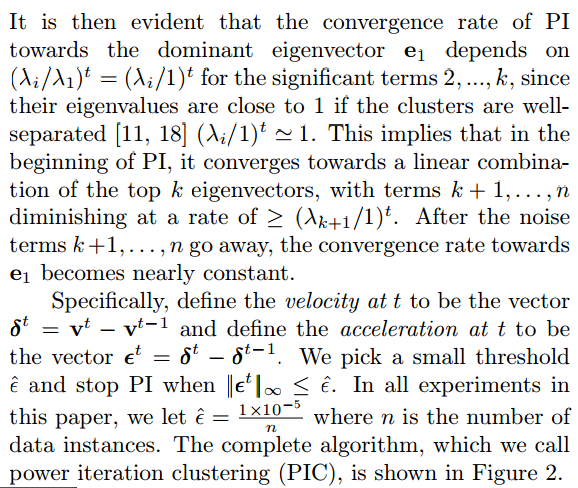从PIC整个计算流程可以看出，PIC的计算效率高，因为它只涉及到矩阵的向量迭代乘法和原始数据嵌入后的低维聚类，尤其是对大型稀疏矩阵，向量迭代乘法的计算量更小。

# Spark MLlib 代码

应该说Spark中Graphx 模块的发展，极大促进了基于图计算的诸多算法在大数据集上的实现（关于Graphx的内容，也可以参考我Spark系列的博文），谱聚类方法本身就是一种基于图的技术，幂迭代聚类和谱聚类一样，也是基于图的聚类技术，都是先把获得的原始数据集抽象为图结构后（顶点，边和三元组等等）才进一步进行分析的。

程序关键参数是确保输入的图RDD符合无向，非负等多个要求，这在下面程序注释中都已经一一说明了。
另外K值的选取和k-means方法一样，仍然是一个难题，可以参看一下我Spark系列文章k-means那篇。

import org.apache.spark.sql.SparkSession
import org.apache.log4j.{Level, Logger}
import org.apache.spark.SparkContext
import org.apache.spark.rdd.RDD
import org.apache.spark.mllib.clustering.PowerIterationClustering

object myClusters2 {

//产生一个分布在圆形上的数据模型（见程序后面的图）：参数为半径和点数
def generateCircle(radius: Double, n: Int): Seq[(Double, Double)] = {
Seq.tabulate(n) { i =>
val theta = 2.0 * math.Pi * i / n
}
}

//产生同心圆样的数据模型（见程序后面的图）：参数为同心圆的个数和点数
//第一个同心圆上有nPoints个点，第二个有2*nPoints个点，第三个有3*nPoints个点，以此类推
def generateCirclesRdd(
sc: SparkContext,
nCircles: Int,
nPoints: Int): RDD[(Long, Long, Double)] = {
val points = (1 to nCircles).flatMap { i =>
generateCircle(i, i * nPoints)
}.zipWithIndex
val rdd = sc.parallelize(points)
val distancesRdd = rdd.cartesian(rdd).flatMap { case (((x0, y0), i0), ((x1, y1), i1)) =>
if (i0 < i1) {
Some((i0.toLong, i1.toLong, gaussianSimilarity((x0, y0), (x1, y1))))
} else {
None
}
}
distancesRdd
}

/**
* Gaussian Similarity
*/
def gaussianSimilarity(p1: (Double, Double), p2: (Double, Double)): Double = {
val ssquares = (p1._1 - p2._1) * (p1._1 - p2._1) + (p1._2 - p2._2) * (p1._2 - p2._2)
math.exp(-ssquares / 2.0)
}

def main(args:Array[String]){

//屏蔽日志
Logger.getLogger("org.apache.spark").setLevel(Level.ERROR)
Logger.getLogger("org.eclipse.jetty.server").setLevel(Level.OFF)

val warehouseLocation = "/Spark/spark-warehouse"

val spark=SparkSession
.builder()
.appName("myClusters")
.master("local")
.config("spark.sql.warehouse.dir",warehouseLocation)
.getOrCreate();

val sc=spark.sparkContext

// 产生测试数据
val circlesRdd = generateCirclesRdd(sc, 2, 4)//产生具有2个半径不同的同心圆模型的相似度矩阵，第一个圆上有4个点，第二个圆上有2*4=8个点
//circlesRdd.take(100).foreach(p=>print(p+"\n"))
/**
* circlesRdd数据格式实例：(srcId, dstId, similarity)
* (0,1,0.22313016014842987)
* (0,2,0.22313016014842973)
* (1,2,0.22313016014842987)
*/

/**PowerIterationClustering函数输入输出说明
*
*  It takes an RDD of (srcId, dstId, similarity) tuples and outputs a model
*  with the clustering assignments. The similarities must be nonnegative.
*  PIC assumes that the similarity measure is symmetric. A pair (srcId, dstId)
*  regardless of the ordering should appear at most once in the input data.
*  If a pair is missing from input, their similarity is treated as zero.
*  它的输入circlesRdd是(srcId, dstId,similarity) 三元组的RDD（起始点->终点，相似度为边权重）。
*  显然算法中选取的相似度函数是非负的，而且PIC 算法假设相似函数还满足对称性（边权重代表相似度，非负）。
*  输入数据中（srcId,dstId）对不管先后顺序如何只能出现至多一次（即是无向图，两顶点边权重是唯一的）
*  如果输入数据中不含这个数据对（不考虑顺序），则这两个数据对的相似度为0（没有这条边）.
*
*/
val modelPIC = new PowerIterationClustering()
.setK(2)// k : 期望聚类数
.setMaxIterations(40)//幂迭代最大次数
.setInitializationMode("degree")//模型初始化，默认使用”random” ，即使用随机向量作为初始聚类的边界点，可以设置”degree”（就是图论中的度）。
.run(circlesRdd)

//输出聚类结果
val clusters = modelPIC.assignments.collect().groupBy(_.cluster).mapValues(_.map(_.id))
val assignments = clusters.toList.sortBy { case (k, v) => v.length }
val assignmentsStr = assignments
.map { case (k, v) =>
s"$k ->${v.sorted.mkString("[", ",", "]")}"
}.mkString(", ")
val sizesStr = assignments.map {
_._2.length
}.sorted.mkString("(", ",", ")")
println(s"Cluster assignments: $assignmentsStr\ncluster sizes:$sizesStr")
/*
* Cluster assignments: 1 -> [4,6,8,10], 0 -> [0,1,2,3,5,7,9,11]
* cluster sizes: (4,8)
*/

}

}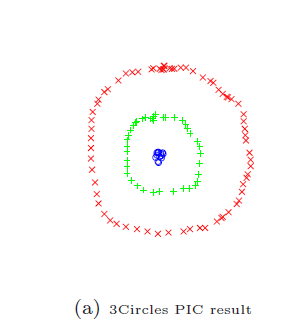（1）Spark官网
（2)《power iteration clustering》 Lin and Cohen
（3）Spectral Clustering via the Power Method – Provably
（4）百度文库 xiaofei id
（5）维基 https://en.wikipedia.org/wiki/Power_iteration
（6）幂法求矩阵特征值的一些补充 何 明 安徽大学计算机科学与工程系 合肥 230039

09-26
05-04594002-1049
05-152万+
09-212万+
04-141万+
09-121102
03-141233
01-13576
09-26
10-21475
11-032564
09-251万+
06-092884
01-162546
06-226946
05-152万+
04-23623点击重新获取扫码支付余额充值